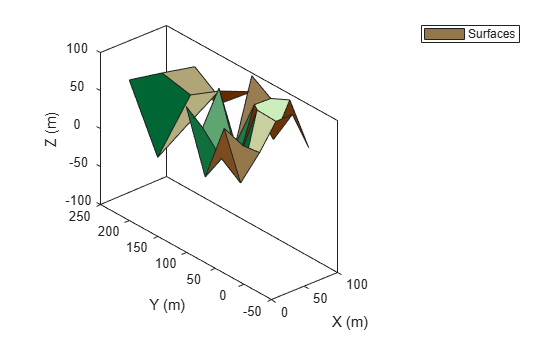# surfacePlotter

Create surface plotter

Since R2022b

## Syntax

``sPlotter = surfacePlotter(tp)``
``sPlotter = surfacePlotter(tp,Name=Value)``

## Description

````sPlotter = surfacePlotter(tp)` creates a `SurfacePlotter` object for use with a `theaterPlot` object `tp`. Use the `plotSurface` function to plot surfaces using the `SurfacePlotter` object.```

example

````sPlotter = surfacePlotter(tp,Name=Value)` creates a `SurfacePlotter` object with additional options specified by one or more name-value arguments. For example, `surfacePlotter(DisplayName="Surfaces")` specifies `Surfaces` as the name displayed in the legend.```

## Examples

collapse all

`scenario = radarScenario;`

Define the terrain and boundaries of two surfaces and add the two surfaces to the radar scenario.

```terrain1 = randi(100,4,5); terrain2 = randi(100,3,3); boundary1 = [0 100; 0 100-eps]; boundary2 = [0 100; 100 200]; s1 = landSurface(scenario,Terrain=terrain1,Boundary=boundary1); s2 = landSurface(scenario,Terrain=terrain2,Boundary=boundary2);```

Obtain the plotter data by using the `surfacePlotterData` function.

`plotterData = surfacePlotterData(scenario.SurfaceManager)`
```plotterData=1×2 struct array with fields: X Y Z C ```

Create a `theaterPlot` object and specify the axis limits of the plot.

`theaterpplot = theaterPlot(ZLimits=[-50 150],YLimits=[-50 250],ZLimits=[-100 100]);`

Create a surface plotter.

`plotter = surfacePlotter(theaterpplot,DisplayName="Surfaces");`

Plot surfaces in the theater plot. Change view angles for better visualization.

```plotSurface(plotter,plotterData) view(-41,29)```## Input Arguments

collapse all

Theater plot, specified as a `theaterPlot` object.

### Name-Value Arguments

Specify optional pairs of arguments as `Name1=Value1,...,NameN=ValueN`, where `Name` is the argument name and `Value` is the corresponding value. Name-value arguments must appear after other arguments, but the order of the pairs does not matter.

Before R2021a, use commas to separate each name and value, and enclose `Name` in quotes.

Example: `DisplayName="GroundSurface"`

Plot name to display in legend, specified as a character vector or string scalar. If you do not specify this argument, the function does not display a plot name.

Tag associated with the plotter, specified as a character vector or string. You can use the `findPlotter` function to identify plotters based on their tag. The default value is `'PlotterN'`, where N is an integer that corresponds to the Nth plotter associated with the `theaterPlot`.

Face alpha value for all plotted surfaces, specified as a scalar in the range `[0 1]`.

Edge color for all plotted surfaces, specified as a character vector of a valid color, a string scalar of a valid color, an RGB triplet, or a hexadecimal color code.

## Output Arguments

collapse all

Surface plotter, returned as a `SurfacePlotter` object. You can modify this object by changing its property values. The property names correspond to the name-value arguments of the `surfacePlotter` function.

To plot surfaces, use the `plotSurface` function.

## Version History

Introduced in R2022b